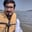Related Tags

python
pandas
columns
communitycreator

# How to normalize all columns in a dataframe in pandasArslan Bajwa

In Python, the pandas library includes built-in functionalities that allow you to perform different tasks with only a few lines of code. One of these functionalities is the normalization of all columns in a dataframe.

## Method

To normalize all columns of the dataframe, we first subtract the column mean, and then divide by the standard deviation.

#importing pandas and numpy libraries
import pandas as pd
import numpy as np

#initializing pandas dataframe with random values
df = pd.DataFrame(np.random.randint(1,100, 50).reshape(5, -1))

#normalizing dataframe
result = df.apply(lambda iterator: ((iterator - iterator.mean())/iterator.std()).round(2))

print(result)


Then, we range all columns of the dataframe, such that the min is 0 and the max is 1.

#importing pandas and numpy libraries
import pandas as pd
import numpy as np

#initializing pandas dataframe with random values
df = pd.DataFrame(np.random.randint(1,100, 50).reshape(5, -1))

#normalizing dataframe
result = df.apply(lambda iterator: ((iterator.max() - iterator)/(iterator.max() - iterator.min())).round(2))

print(result)  

RELATED TAGS

python
pandas
columns
communitycreator

CONTRIBUTORArslan Bajwa
RELATED COURSES

View all Courses

Keep Exploring

Learn in-demand tech skills in half the time